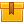# Parallel Circuits

## Prerequisites

Understanding Ohm's Law, Kirchhoff??s Law, and series circuits is recommended.

## Description

This is the fourth lesson in the iKNOW Electrical Theory for Troubleshooters Library. This lesson describes the behavior of voltage, current, and resistance in a parallel circuit. The learner is also instructed in the identification of the series and parallel portions of a series-parallel circuit.

## Objectives

Describe how voltage and current behave in a parallel circuit. Describe how resistance behaves in a parallel circuit. Identify the series portions of a series-parallel circuit. Identify the parallel portions of a series-parallel circuit. Simplify a series-parallel circuit to determine how voltage, current, and resistance behave.

## ContentThis is the fourth lesson in the iKNOW™ Electrical Theory for
Troubleshooters Library. This lesson describes the behavior of voltage,
current, and resistance in a parallel circuit. The learner is also
instructed in the identification of the series and parallel portions of a
series-parallel circuit.

•Parallel Circuits

0
0 Reviews